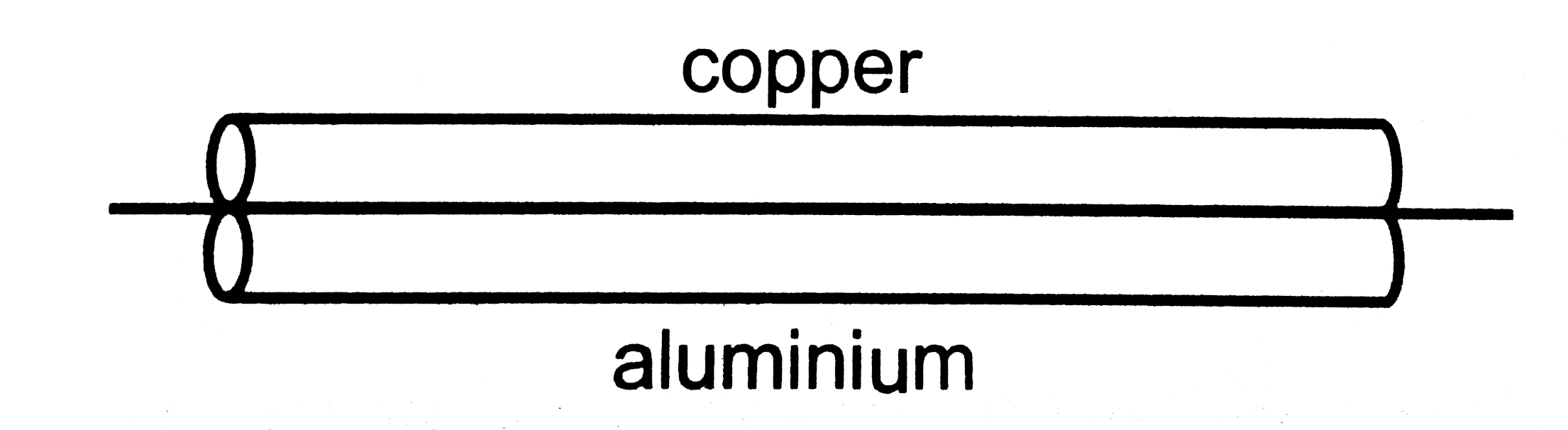# A copper rod of length 20cm and cross-sectional area 2mm2is joined with a similar aluminium rod as shown in figure .Find the resistance of the combination between the ends, Resistivity of copper =1.7×10−8(Ω)mand that of aluminium=2.6×10−8(Ω)m.Khareedo DN Pro and dekho sari videos bina kisi ad ki rukaavat ke!

##### Updated On: 27-06-2022

Text Solution

Solution
The resistance of the copper rod
(ρ)lA=(1.7×108(Ω)m)×(20×102m)2.0×106m2
=1.7×103(Ω).
Similarly, the resistance of the aluminium rid =2.6×103(Ω).
these rods are joined in parallel so that the equivalent risistance R between the ends is given by
1R=11.7×103(Ω)+12.6×103(Ω).
or, R=(1.7xx2.6)/(4.3)xx10^(-3)(Omega)~~1.0m(Omega).